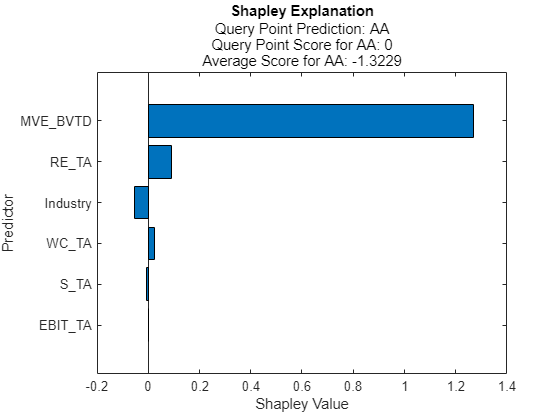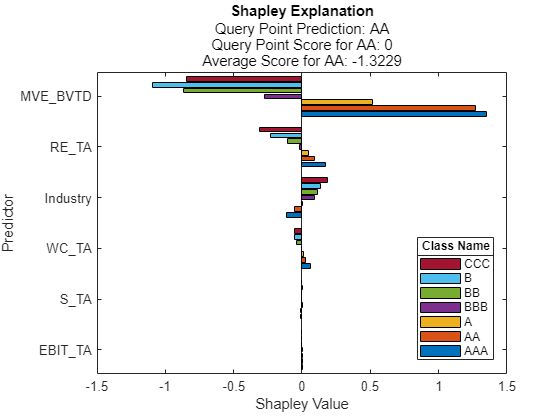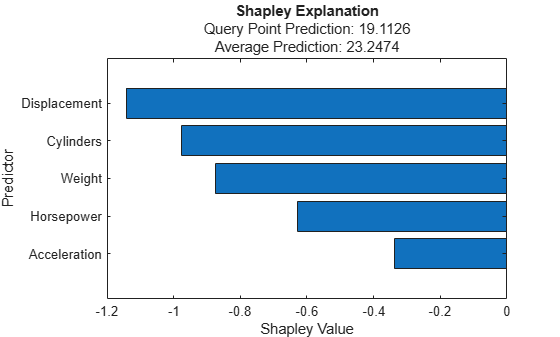# plot

Plot Shapley values

Since R2021a

## Syntax

``plot(explainer)``
``plot(explainer,Name,Value)``
``plot(ax,___)``
``b = plot(___)``

## Description

example

````plot(explainer)` creates a horizontal bar graph of the Shapley values of the `shapley` object `explainer`. These values are stored in the object's `ShapleyValues` property. Each bar shows the Shapley value of each feature in the blackbox model (`explainer.BlackboxModel`) for the query point (`explainer.QueryPoint`).```

example

````plot(explainer,Name,Value)` specifies additional options using one or more name-value arguments. For example, specify `'NumImportantPredictors',5` to plot the Shapley values of the five features with the highest absolute Shapley values.```
````plot(ax,___)` displays the plot in the target axes `ax`. Specify the axes as the first argument in any of the previous syntaxes. (since R2023b)```
````b = plot(___)` returns a bar graph object `b` using any of the input argument combinations in the previous syntaxes. Use `b` to query or modify Bar Properties of the bar graph after it is created.```

## Examples

collapse all

Train a classification model and create a `shapley` object. Then plot the Shapley values by using the object function `plot`.

Load the `CreditRating_Historical` data set. The data set contains customer IDs and their financial ratios, industry labels, and credit ratings.

`tbl = readtable('CreditRating_Historical.dat');`

Display the first three rows of the table.

`head(tbl,3)`
``` ID WC_TA RE_TA EBIT_TA MVE_BVTD S_TA Industry Rating _____ _____ _____ _______ ________ _____ ________ ______ 62394 0.013 0.104 0.036 0.447 0.142 3 {'BB'} 48608 0.232 0.335 0.062 1.969 0.281 8 {'A' } 42444 0.311 0.367 0.074 1.935 0.366 1 {'A' } ```

Train a blackbox model of credit ratings by using the `fitcecoc` function. Use the variables from the second through seventh columns in `tbl` as the predictor variables. A recommended practice is to specify the class names to set the order of the classes.

```blackbox = fitcecoc(tbl,'Rating', ... 'PredictorNames',tbl.Properties.VariableNames(2:7), ... 'CategoricalPredictors','Industry', ... 'ClassNames',{'AAA' 'AA' 'A' 'BBB' 'BB' 'B' 'CCC'});```

Create a `shapley` object that explains the prediction for the last observation. For faster computation, subsample 25% of the observations from `tbl` with stratification and use the samples to compute the Shapley values.

`queryPoint = tbl(end,:)`
```queryPoint=1×8 table ID WC_TA RE_TA EBIT_TA MVE_BVTD S_TA Industry Rating _____ _____ _____ _______ ________ ____ ________ ______ 73104 0.239 0.463 0.065 2.924 0.34 2 {'AA'} ```
```rng('default') % For reproducibility c = cvpartition(tbl.Rating,'Holdout',0.25); tbl_s = tbl(test(c),:); explainer = shapley(blackbox,tbl_s,'QueryPoint',queryPoint);```

For a classification model, `shapley` computes Shapley values using the predicted class score for each class. Display the values in the `ShapleyValues` property.

`explainer.ShapleyValues`
```ans=6×8 table Predictor AAA AA A BBB BB B CCC __________ _________ __________ ___________ __________ ___________ __________ __________ "WC_TA" 0.051044 0.022644 0.0096138 0.0015955 -0.027857 -0.041342 -0.039476 "RE_TA" 0.16729 0.094791 0.05308 -0.011178 -0.08769 -0.20847 -0.29204 "EBIT_TA" 0.0012014 0.00053339 0.00043344 0.00012321 -0.00066993 -0.0013388 -0.0011793 "MVE_BVTD" 1.3377 1.338 0.67839 -0.027654 -0.55142 -0.75321 -0.59576 "S_TA" -0.012482 -0.009097 -0.00074119 -0.0035582 -7.3517e-05 0.0014497 -0.0020609 "Industry" -0.099094 -0.046871 0.0031376 0.080071 0.089726 0.099687 0.15691 ```

The `ShapleyValues` property contains the Shapley values of all features for each class.

Plot the Shapley values for the predicted class by using the `plot` function.

`plot(explainer)`The horizontal bar graph shows the Shapley values for all variables, sorted by their absolute values. Each Shapley value explains the deviation of the score for the query point from the average score of the predicted class, due to the corresponding variable.

Plot the Shapley values for all classes by specifying all class names in `explainer.BlackboxModel`.

`plot(explainer,'ClassNames',explainer.BlackboxModel.ClassNames)`Train a regression model and create a `shapley` object. Use the object function `fit` to compute the Shapley values for the specified query point. Then plot the Shapley values of the predictors by using the object function `plot`. Specify the number of important predictors to plot when you call the `plot` function.

Load the `carbig` data set, which contains measurements of cars made in the 1970s and early 1980s.

`load carbig`

Create a table containing the predictor variables `Acceleration`, `Cylinders`, and so on, as well as the response variable `MPG`.

`tbl = table(Acceleration,Cylinders,Displacement,Horsepower,Model_Year,Weight,MPG);`

Removing missing values in a training set can help reduce memory consumption and speed up training for the `fitrkernel` function. Remove missing values in `tbl`.

`tbl = rmmissing(tbl);`

Train a blackbox model of `MPG` by using the `fitrkernel` function

```rng('default') % For reproducibility mdl = fitrkernel(tbl,'MPG','CategoricalPredictors',[2 5]);```

Create a `shapley` object. Specify the data set `tbl`, because `mdl` does not contain training data.

`explainer = shapley(mdl,tbl)`
```explainer = shapley with properties: BlackboxModel: [1x1 RegressionKernel] QueryPoint: [] BlackboxFitted: [] ShapleyValues: [] X: [392x7 table] CategoricalPredictors: [2 5] Method: 'interventional-kernel' Intercept: 22.6202 NumSubsets: 64 ```

`explainer` stores the training data `tbl` in the `X` property.

Compute the Shapley values of all predictor variables for the first observation in `tbl`.

`queryPoint = tbl(1,:)`
```queryPoint=1×7 table Acceleration Cylinders Displacement Horsepower Model_Year Weight MPG ____________ _________ ____________ __________ __________ ______ ___ 12 8 307 130 70 3504 18 ```
`explainer = fit(explainer,queryPoint);`

For a regression model, `shapley` computes Shapley values using the predicted response, and stores them in the `ShapleyValues` property. Display the values in the `ShapleyValues` property.

`explainer.ShapleyValues`
```ans=6×2 table Predictor ShapleyValue ______________ ____________ "Acceleration" -0.1561 "Cylinders" -0.18306 "Displacement" -0.34203 "Horsepower" -0.27291 "Model_Year" -0.2926 "Weight" -0.32402 ```

Plot the Shapley values for the query point by using the `plot` function. Specify `'NumImportantPredictors',5` to plot only the five most important predictors for the predicted response.

`plot(explainer,'NumImportantPredictors',5)`The horizontal bar graph shows the Shapley values for the five most important predictors, sorted by their absolute values. Each Shapley value explains the deviation of the prediction for the query point from the average, due to the corresponding variable.

## Input Arguments

collapse all

Object explaining the blackbox model, specified as a `shapley` object.

Since R2023b

Axes for the plot, specified as an `Axes` object. If you do not specify `ax`, then `plot` creates the plot using the current axes. For more information on creating an `Axes` object, see `axes`.

### Name-Value Arguments

Specify optional pairs of arguments as `Name1=Value1,...,NameN=ValueN`, where `Name` is the argument name and `Value` is the corresponding value. Name-value arguments must appear after other arguments, but the order of the pairs does not matter.

Before R2021a, use commas to separate each name and value, and enclose `Name` in quotes.

Example: `plot(explainer,'NumImportantPredictors',5,'ClassNames',c)` creates a bar graph containing the Shapley values of the five most important predictors for the class `c`.

Number of important predictors to plot, specified as a positive integer. The `plot` function plots the Shapley values of the specified number of predictors with the highest absolute Shapley values.

Example: `'NumImportantPredictors',5` specifies to plot the five most important predictors. The `plot` function determines the order of importance by using the absolute Shapley values.

Data Types: `single` | `double`

Class labels to plot, specified as a categorical or character array, logical or numeric vector, or cell array of character vectors. The values and data types in the `'ClassNames'` value must match those of the class names in the `ClassNames` property of the machine learning model in `explainer` (`explainer.BlackboxModel.ClassNames`).

You can specify one or more labels. If you specify multiple class labels, the function plots multiple bars for each feature with different colors.

The default value is the predicted class for the query point (the `BlackboxFitted` property of `explainer`).

This argument is valid only when the machine learning model (`BlackboxModel`) in `explainer` is a classification model.

Example: `'ClassNames',{'red','blue'}`

Example: `'ClassNames',explainer.BlackboxModel.ClassNames` specifies `'ClassNames'` as all classes in `BlackboxModel`.

Data Types: `single` | `double` | `logical` | `char` | `cell` | `categorical`

collapse all

### Shapley Values

In game theory, the Shapley value of a player is the average marginal contribution of the player in a cooperative game. In the context of machine learning prediction, the Shapley value of a feature for a query point explains the contribution of the feature to a prediction (response for regression or score of each class for classification) at the specified query point.

The Shapley value of a feature for a query point is the contribution of the feature to the deviation from the average prediction. For a query point, the sum of the Shapley values for all features corresponds to the total deviation of the prediction from the average. That is, the sum of the average prediction and the Shapley values for all features corresponds to the prediction for the query point.

For more details, see Shapley Values for Machine Learning Model.

 Lundberg, Scott M., and S. Lee. "A Unified Approach to Interpreting Model Predictions." Advances in Neural Information Processing Systems 30 (2017): 4765–774.

 Aas, Kjersti, Martin Jullum, and Anders Løland. "Explaining Individual Predictions When Features Are Dependent: More Accurate Approximations to Shapley Values." Artificial Intelligence 298 (September 2021).

 Lundberg, Scott M., G. Erion, H. Chen, et al. "From Local Explanations to Global Understanding with Explainable AI for Trees." Nature Machine Intelligence 2 (January 2020): 56–67.